﻿ 3.4 Pricing Relative to the Yield Curveoffice (412) 967-9367
fax (412) 967-5958
toll-free 1 (800) 214-3480

3.4  American Put Option:  Zero-Dividend Case

 Y

ou have found that an American call option should not be exercised prior to maturity.  What is the case for the American put option? Starting again with the one-period risk-neutral valuation model for a put option, we will try to obtain a similar bound for the put option as we did for the call option.  The risk-neutral valuation formula says that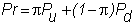where  p is the risk-neutral probability (see topic 2.7, Risk-Neutral Valuation Principle in Chapter 2), and r is one plus the interest rate.  In the one-period model Pu and Pd are the terminal put option values.  In other words, Pu = max{X-Su,0} and Pd = max{X-Sd,0}.

Substituting these values, we get: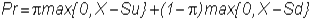and adding and subtracting X in the right-hand side of the equation yields: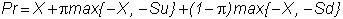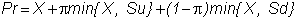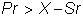which implies P > X/r - S since r > 1.

But what is the put option worth if you exercise it?

If you exercise the put option, you sell a stock worth S and receive the strike price, X.  The exercise value is thus X - S, and since r > 1 it is possible that the exercise value of the put option is greater than the value if you hold onto it:This is so when S is very close to zero, so P is approximately X/r, and thus early exercise is always desirable since r > 1.

Therefore, the right to early exercise may have value for an American put option.

Unfortunately, there is no formula that gives you the price of an American put option.  Various numerical methods have been developed to calculate its value.  One such method is based on the binomial model, where you check at every node whether the option should be exercised.

Another variable which affects the decision to exercise an option early is the dividend paid by the stock.  We deal with this next in the topic  Dividends and American Options.